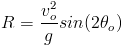# Practice Test

The following test will help solidify the concepts which you have just reviewed.

Personal Information

This information will allow us to send your score to you.

1 a.) A particle travels 15 meters along the posative x axis in 3 seconds, then reverses direction and travels 5 meters in 2 seconds. What is the average velocity over the 5 second time interval?
3
10
2
4
1 b.) What are the units on this measurment?
2.) Which of the following are vector quantities?
Mass
Force
Velocity
Work
Weight
Acceleration
3.) An arrow is fired at an initial velocity vo from the top of a 100 meter tall cliff at an initial angle θ. Explain in the space below why the range equation cannot be used to calculate the distance which the arrow travels.

The range equation:4.) Three forces act on a 50 kg box which rests on a frictionless surface. The first force points in the posative x direction with a megnitude of 6N. The second force points in the negative x direction with a megnitude of 4N. The third force points in the negative x direction with a megnitude of 12N. What is the magnitude and direction of the block's acceleration?
5 m/s2 in the posative x direction
8.3 m/s2 in the posative x direction
5 m/s2 in the negative x direction
25 m/s2 in the negative x direction
5.) If a 20 kg particle has 1.690 kJ of kinetic energy, how fast is the particle moving?
169 m/s
13 m/s
9.19 m/s
.411 m/s
6.) A particle is dropped from a height of 40 meters how fast is the particle moving after it has fallen 40 meters? (you may use energy or kinematics to solve this question)
56 m/s
14 m/s
784 m/s
28 m/s
not enough information is given to solve this question